• +91 9971497814
• info@interviewmaterial.com

# Chapter 13 Limits and Derivatives Ex-13.2 Interview Questions Answers

### Related Subjects

Question 1 : Find the derivative of x2– 2 at x = 10

Let f (x) = x2 –2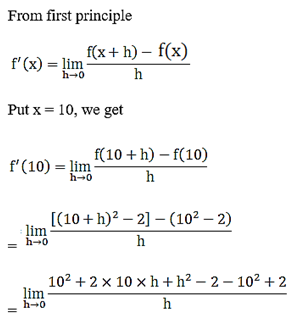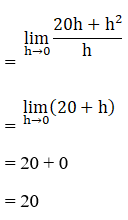Question 2 : Find the derivative of x at x = 1.

Let f (x) = x
Then,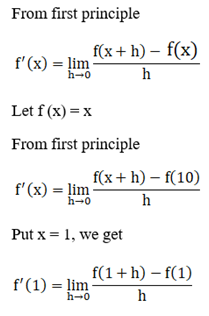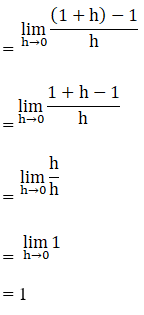Question 3 : Find the derivative of 99x at x = l00.

Let f (x) = 99x,
From first principle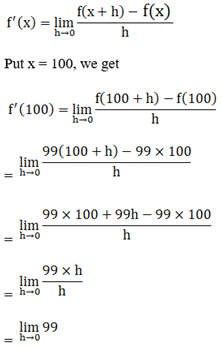=99

Question 4 :

Find the derivativeof the following functions from first principle

(i) x3 –27

(ii) (x – 1) (x – 2)

(iii) 1 / x2

(iv) x + 1 / x – 1

(i) Let f (x) = x3 –27

From first principle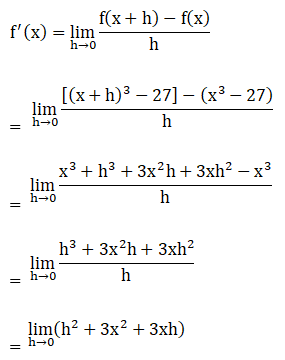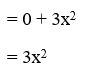(ii) Let f (x) = (x – 1) (x – 2)

From first principle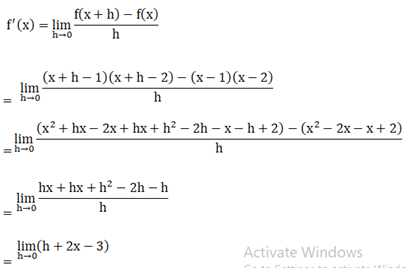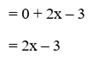(iii) Let f (x) = 1 / x2

From first principle, we get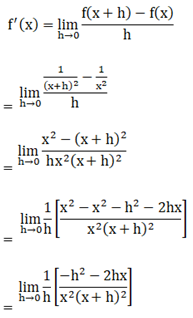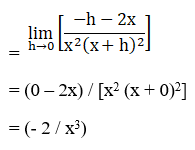(iv) Let f (x) = x + 1 / x – 1

From first principle, we get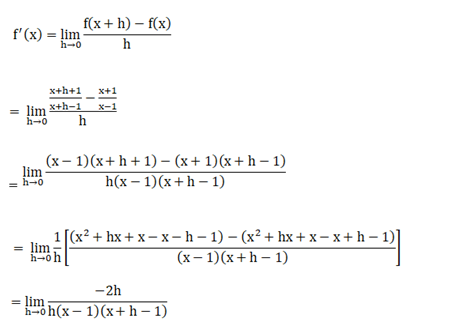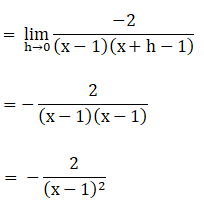Question 5 : For the function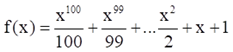.Prove that f’ (1) =100 f’ (0).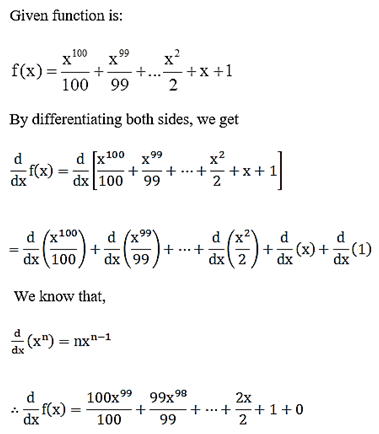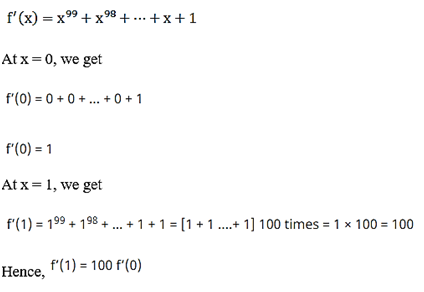Question 6 : Find the derivative of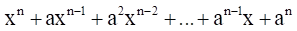for some fixed real number a.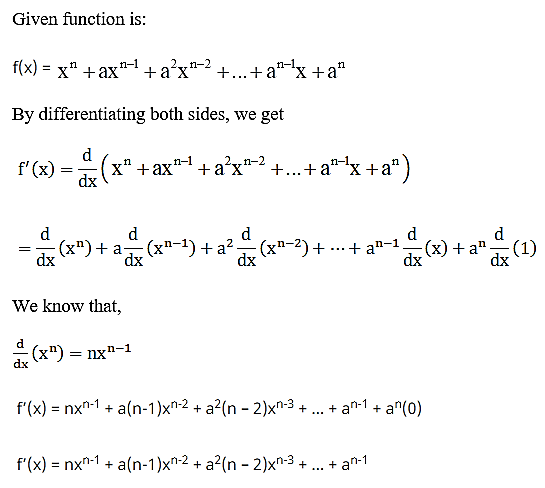Question 7 :

For some constantsa and b, find the derivative of
(i) (x − a) (x − b)

(ii) (ax2 +b)2

(iii) x – a / x – b

(i) (x – a) (x – b)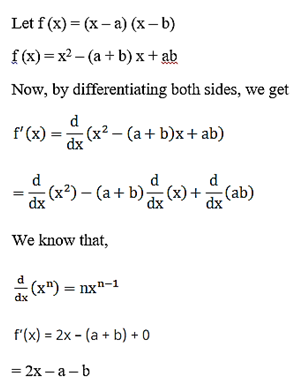(ii) (ax2 + b)2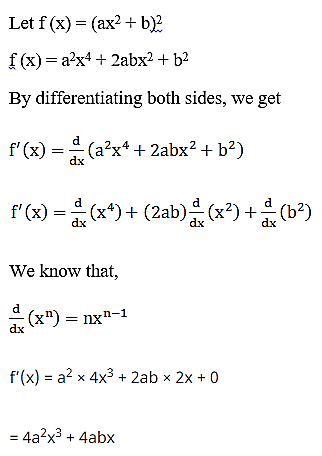= 4ax (ax2 + b)

(iii) x – a / x – b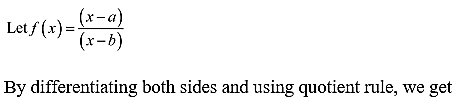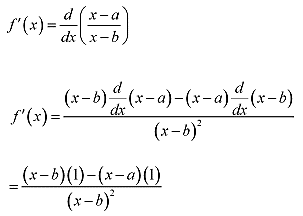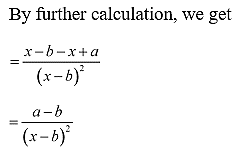Question 8 : Find the derivative of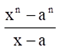for some constant a.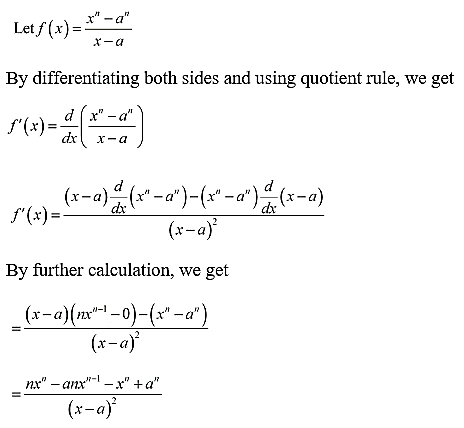Question 9 :

Find the derivativeof

(i) 2x – 3 / 4

(ii) (5x3 +3x – 1) (x – 1)

(iii) x-3 (5+ 3x)

(iv) x5 (3– 6x-9)

(v) x-4 (3– 4x-5)

(vi) (2 / x + 1) – x2 / 3x – 1